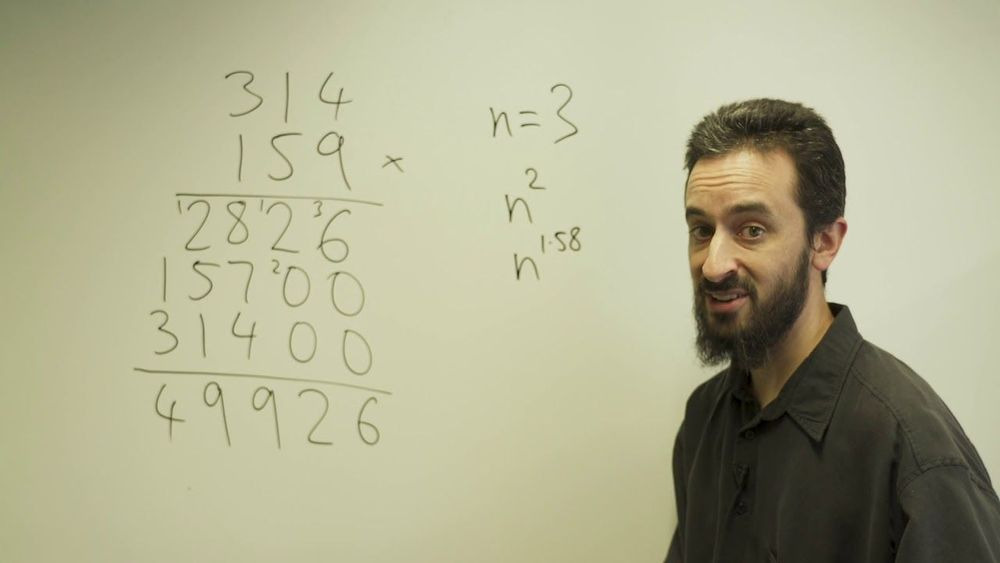Menu

## BlogA pair of mathematicians from Australia and France have devised an alternative way to multiply numbers together, while solving an algorithmic puzzle that has perplexed some of the greatest math minds for almost half a century.

For most of us, the way we multiply relatively small numbers is by remembering our times tables – an incredibly handy aid first pioneered by the Babylonians some 4,000 years ago.

But what if the numbers get bigger? Well, if the figures get unwieldy – and assuming we don’t have a calculator or computer, of course – most of us would then turn to long multiplication: another useful trick we learn in school, and a trusty technique for multiplying basically any two numbers together.